# Introduction to ggplot2 and to ggtree

Hi everyone,

That’s the first tutorial I write, I will assume you know some basis of R. I try to write more to improve my writing for my PhD and for myself as English is not my native language. So please give me your feedback. The easiest way to contact me is via Twitter or via email.

In this blog post I will start with a quick introduction to ggplot2, then show how to visualise a simple tree with ggtree1.

In order to properly run the commands of this introduction in R you will need the following packages:

``````library(ggplot2) # install.packages("ggplot2")
library(dplyr) # install.packages("dplyr")
library(ggtree) # source("https://bioconductor.org/biocLite.R"); biocLite("ggtree")
``````

## ggplot2

Ggplot2 implements a “grammar of graphics” (gg). As far as I understand it, it’s a unified language to visualise data. This language is made of words, the way this words works together is defined by the grammar, but the words are not limited qualitatively or qualitatively. We we can create our own words, and if we followed the rules, these words will work just fine with others. In ggplot2, these words are “geoms”, “stats”, “scales”, “facets” and “themes”.

For more details and examples, please use the ggplot2 reference. It’s easier to use than the basic documentation you can have access from R with `?ggplot`.

In vanilla ggplot 2 we have geoms like `geom_point` to draw points, `geom_line` to draw lines, etc… But one strength of ggplot is the possibility to use extensions like ggtree, ggforce or cowplot, developed by different persons these packages add new geoms, scales and themes we can use together. For example ggtree adds `geom_tree` (we will take about it later), ggforce adds `geom_bezier` and cowplot is great to make publication ready plots.

Most of the these are coded by different persons but should all work together nicely. It’s something more to learn in addition to base R, but once you understand how it works it become really easy to create nice data visualisation and to integrate them nicely in your analysis pipeline.

The first thing we want to do is to create a ggplot object:

``````ggplot()
``````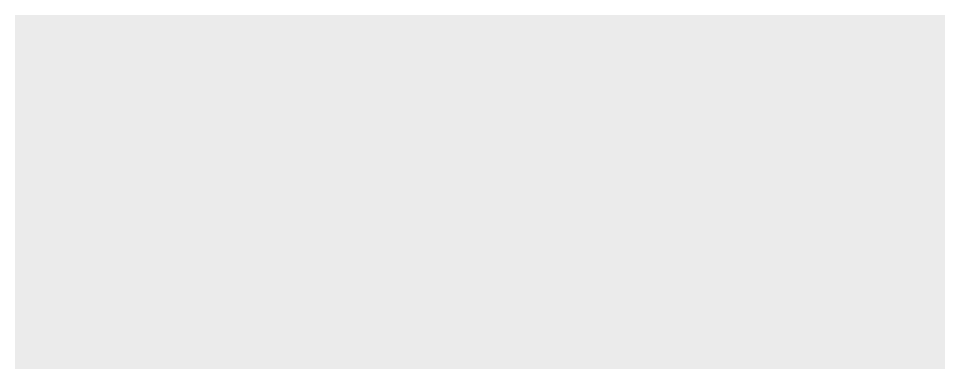Great! A big grey rectangle!

I know it’s not really useful, but every great painter has to start from a blank canvas. The function will plot the things you ask it to plot, the way you ask it to do it and with the data you give it. So far we didn’t give it anything, so it’s just grey.

You can change the background colour2 by modifying the theme of the plot with the function `theme()` or using a predefined theme like `theme_bw()` or `theme_fivethirtyeight()` from the package ggthemes.

``````ggplot() +
theme(panel.background = element_rect(fill = "#56B4E9"))
``````Note: that if you want to add modifications to a predefined theme with `theme()` you need to call it after, otherwise the theme will override your modifications.

Back to our plot.

Let’s say we want to plot data points, we are going to use the geom `geom_point`. For other kind of visualisations we could use different geoms like `geom_density`. One particularity of this geom is that it will compute the density from your data set.

``````ggplot() +
geom_point()
``````Again! The same big grey rectangle! 3

We still haven’t said to ggplot what to plot. We need to define an aesthetic mapping with the function `aes`. If you look at the documentation for `geom_points` with `?geom_point` in the “aesthetics” section it will say that it needs a `x` and `y` value to plot each point. So with `aes` we are going to tell ggplot what is the `x` variable and what is the `y`. That’s the minimum for `geom_point`, other aesthetics are available and other geoms may have other mandatory aesthetics.

Here, I will keep it simple and map x with x and y with y.

``````aes(x = x, y = y)
``````
``````## Aesthetic mapping:
## * `x` -> `x`
## * `y` -> `y`
``````

As you can see there is no error message, even if the `x` and `y` passed as arguments of `aes()` are not defined. If I try to call the variable `x` I have an error message, as expected.

``````x
``````
``````## Error in eval(expr, envir, enclos): object 'x' not found
``````

The interesting thing here is that at this point ggplot didn’t evaluated these variables, it just creates objects. The variables will be evaluated only when we try to print the `ggplot` object. One cool thing we can do is to store this objects in variables for later usage.

``````p <- ggplot() +
geom_point()

mapping_points <- aes(x = x, y = y)
``````

And now let’s talk about data. Data is the key of data visualisation4. Ggplot2 works with tidy data sets. You may need to transform your actual data set into this format. If you are a beginner in data science, bioinformatics (or other fields), you will quickly learn that we spend a huge part of our time manipulating data in order to format it in a practical(-ish) way. It’s very rare to find data sets well organised, for example: most bioinformatics tool have their own output format, some more messier than the others, and one of our first task is to tidy it5.

I like to use `dplyr` and `tidyr` to do this job, they are also part of the tidyverse mentioned earlier, but that’s a personal choice, if you are more at ease with other packages, that works too. I personally think that ggplot forcing us to use a unique data format helps a lot, we may not all like it but, it’s standard everyone can work with.

For the purpose of this simple tutorial I’m just going to use random data. Remember that I previously mapped `x` with `x` and `y` with `y`, so let’s create a a `data_frame` (from dplyr) with these variables and random values from the normal distribution.

``````random_data_frame <- tibble(
x = rnorm(5),
y = rnorm(5)
)
``````
xy
-0.5564012-0.6276572
-0.04861920.7942014
-1.7470504-1.4292353
0.26607450.1663130
0.7222369-1.1874387

We finally have everything, let’s plot something!

To do that, we mix our data set with an aesthetic mapping and a geom. I also added a title to make it a bit fancier.

``````ggplot(
data = random_data_frame, # the data set
mapping = mapping_points # the aesthetic mapping we stored previously
) +
geom_point() + # the geom
labs(
title = "some random points"
) # in addition to the title you can use `labs`to add a subtitle,
````````````    # a caption and name the axis
``````

We can also use the operator `%+%` to mix the objects we stored previously. Why doing that? Let’s say we have a much complex data set, a much complex analysis, and all of that in a script we want to be able to run for different datasets. At this point we may want to programmatically create aesthetic mappings, modify the titles, use multiple geom.

Here I stored my plot in a variable and I will ask ggplot to do the same work but with different datasets. One cool thing about `%+%` is it overrides only what we give it, nothing more. Look at the `labs` call for `p_2` and `p_3`, I only change the subtitle, the title stays the same.

``````p_1 <- p %+%
mapping_points %+%
random_data_frame %+%
labs(
title = "some random points",
subtitle = "5 points"
)

# another data set, with more values, but everything else stay the same
p_2 <- p_1 %+% tibble(
x = rnorm(10),
y = rnorm(10)
) %+%
labs( # I only redefine the subtitle as I want to keep the title
subtitle = "10 points"
)

p_3 <- p_1 %+% tibble( # and again
x = rnorm(30),
y = rnorm(30)
) %+%
labs(
subtitle = "30 points"
)
``````
``````p_1
p_2
p_3
``````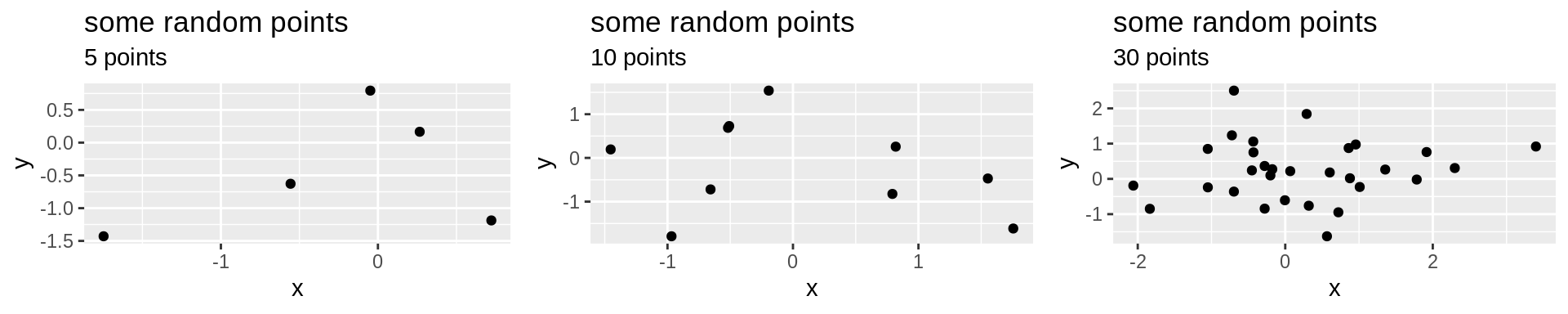I copied and pasted my code to make 3 different plots. But if you use `dplyr` you can for example group your data set with `group_by` then with `summarise` create one or multiple plots for each group. Or if you have data sets nested in a list or a vector, you could use `map` to create a list of plots.

So we have our plots now. I showed you how we can modified them by changing the data set, you can try to change the mapping using `%+%` and a new `aes`. And we can also add elements, it’s not too late. Let’s say that now we want to draw lines between each points, he have to use `geom_line`, we don’t need to change the aesthetic mapping and the data is already stored in the object.

``````p_1 + geom_line(colour = "steelblue")
``````My introduction to ggplot2 comes to an end, if you want to save the plot you can use the `ggsave`function.

``````ggsave(filename = "random_points.pdf") # save the last plot printed
ggsave(filename = "5_random_points.pdf", plot = p_1) # save the plot p_1
``````

For further information I recommend the lecture of the book Hadley Wickham and Garrett Grolemund wrote “R for Data Science”. The ggplot2 reference is also full of examples.

## ggtree

Let’s now move to ggtree!

As part of my PhD I do not only makes plots with lines and points but I also have to manipulate trees. In R trees are stored in objects of the class “phylo”. That’s not really a tidy data set.

``````random_tree <- rtree(5)
random_tree
``````
``````##
## Phylogenetic tree with 5 tips and 4 internal nodes.
##
## Tip labels:
##  "t5" "t2" "t4" "t3" "t1"
##
## Rooted; includes branch lengths.
``````

Ggtree is a package extending the capacities of ggplot2 to work with trees. It contains functions to draw trees, manipulate and annotate them. I will not cover all of these functions here but just show how it works as an extension of ggplot2.

You can find the documentation and examples on Guangchuang Yu’s website, the creator of ggtree, and you use ggtree for your work please cite the paper.

Without using ggtree, but just using what we did with ggplot earlier we can try to plot the tree. Here I’m guessing the name of the variable.

``````ggplot(data = random_tree, mapping = aes(x = x, y = y)) +
geom_point() +
labs(title = "Points from a phylo object")
``````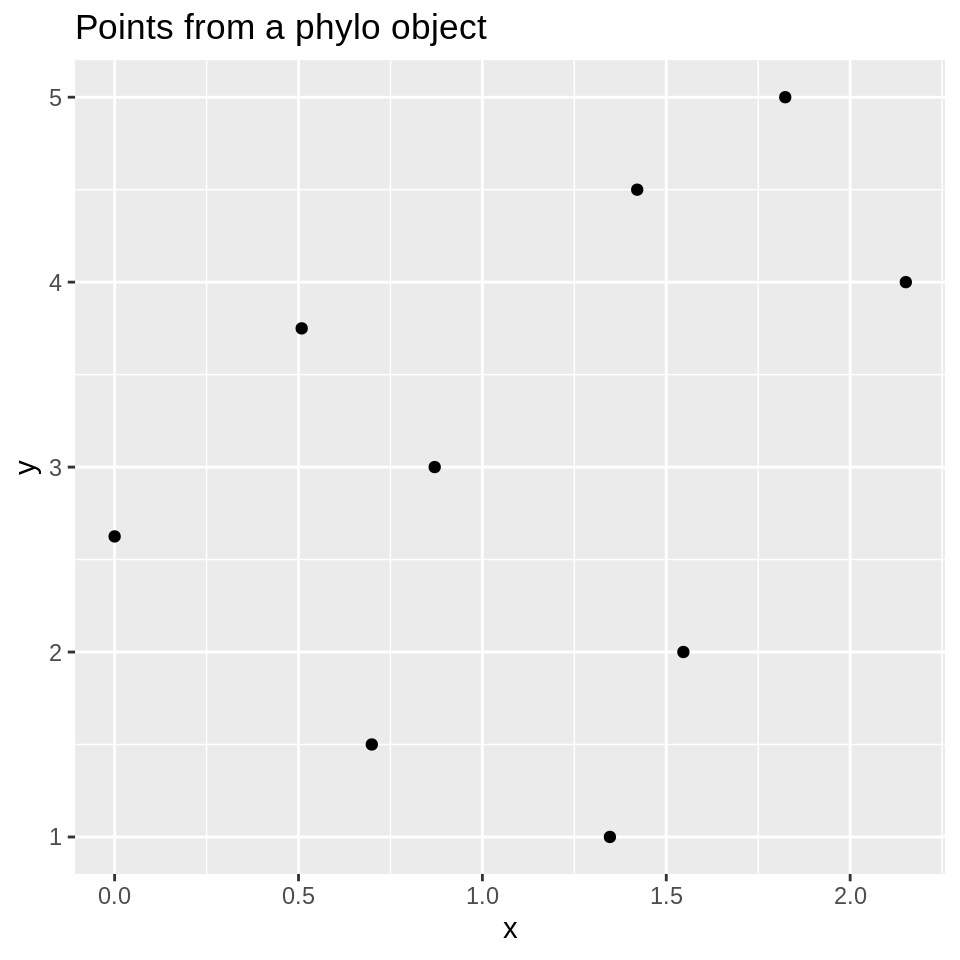It’s not really the tree we were looking for. Indeed, we asked ggplot to draw points so we can’t expect more. We could imagine drawing every lines necessary to make it look like a tree but that would require consequent amount of work and ggtree can do it for us. Ggtree provides `geom_tree`, let’s try it!

``````ggplot(data = random_tree, mapping = aes(x = x, y = y)) +
geom_point() +
geom_tree() +
labs(title = "geom_tree example")
``````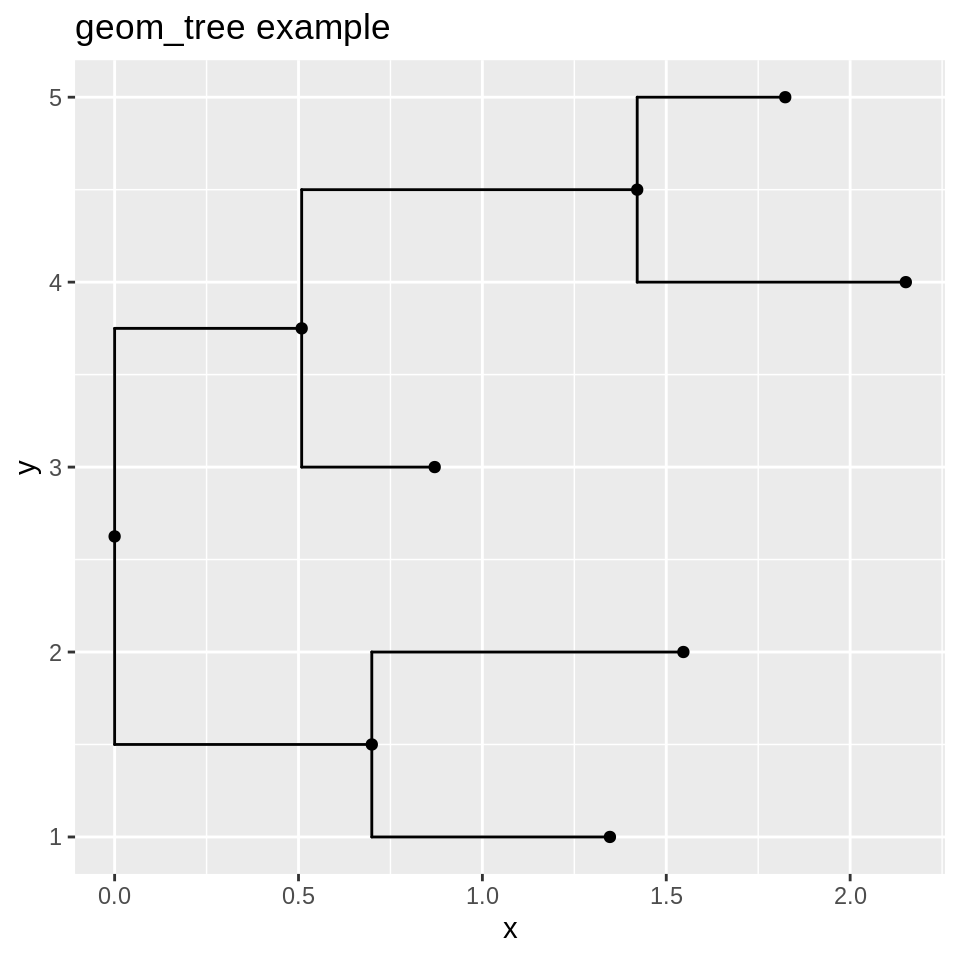It’s not really what we want to use in a scientific article but it allows us to see how it works. Using themes and other geoms we could make it but once again, ggtree already solved this problem, no need to reinvent it. Here, instead of calling `ggplot`we are going to use `ggtree`. It bundles `geom_tree` and everyting to make the tree look nice. In addition we want to display the tip of each leaf of the tree and display the scale of the tree, so we use `geom_tiplab` and `geom_treescale`.

``````ggtree(tr = random_tree) +
geom_tiplab() +
geom_treescale() +
labs(title = "ggtree example")
``````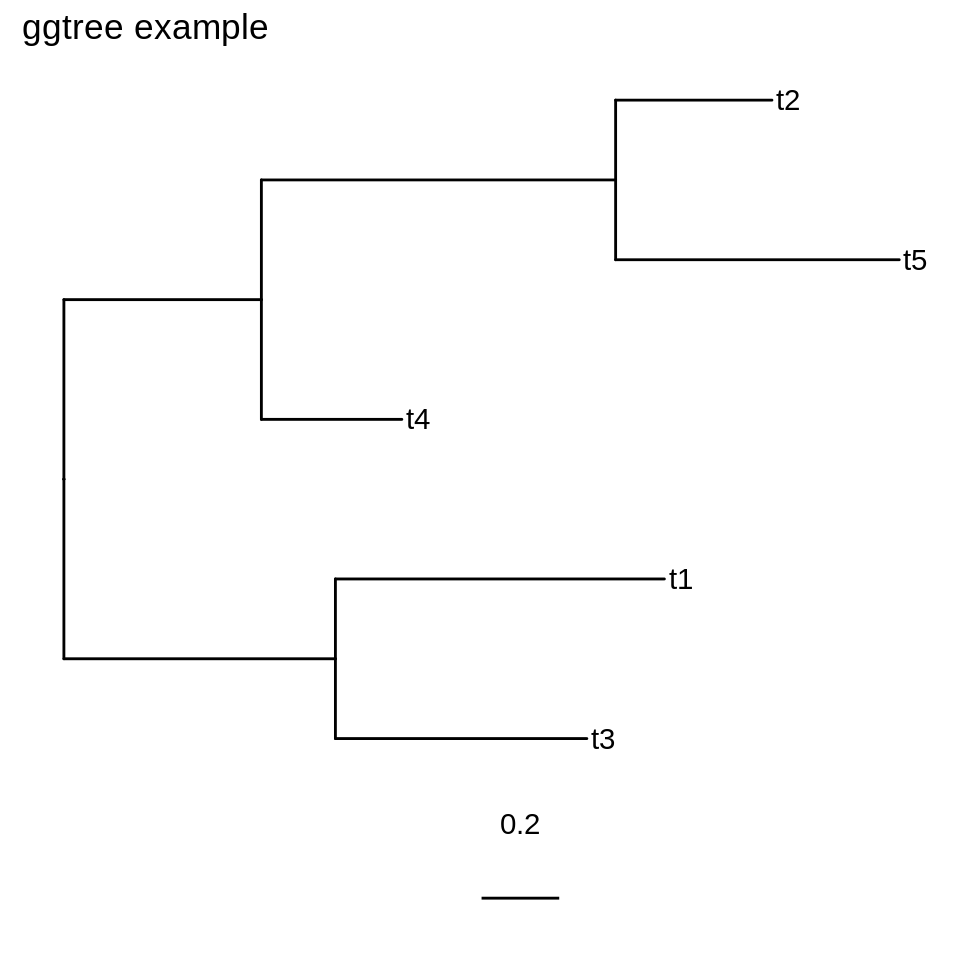As you can see, ggtree took care of the aesthetic mapping and of the geoms.

To better understand how we ggplot and ggtree works with trees, let’s have a look at the tidy version of the phylo object. To do that we use the ggplot’s function `fortify`.

``````fortified_random_tree <- fortify(random_tree)
``````
parentnodebranch.lengthlabelisTipxybranchangle
810.7304323t5TRUE2.15140364.0001.7861875288
820.4025337t2TRUE1.82350505.0001.6222382360
730.3616865t4TRUE0.87031433.0000.6894711216
940.6475054t3TRUE1.34679721.0001.023044672
950.8472369t1TRUE1.54652882.0001.1229103144
660.0000000NAFALSE0.00000002.6250.0000000189
670.5086278NAFALSE0.50862783.7500.2543139270
780.9123435NAFALSE1.42097134.5000.9647996324
690.6992919NAFALSE0.69929191.5000.3496459108

As you can see, each row is a node. The first rows are the leaves and the last ones the internal nodes. Interestingly, we can plot the tree from this data frame. We could easily modify this data frame and then plot a modified tree from it. I will cover this subject in another blog post.

``````ggtree(fortified_random_tree)  +
geom_tiplab() +
geom_treescale() +
labs(title = "ggtree example", subtitle = "using the fortified phylo object")
``````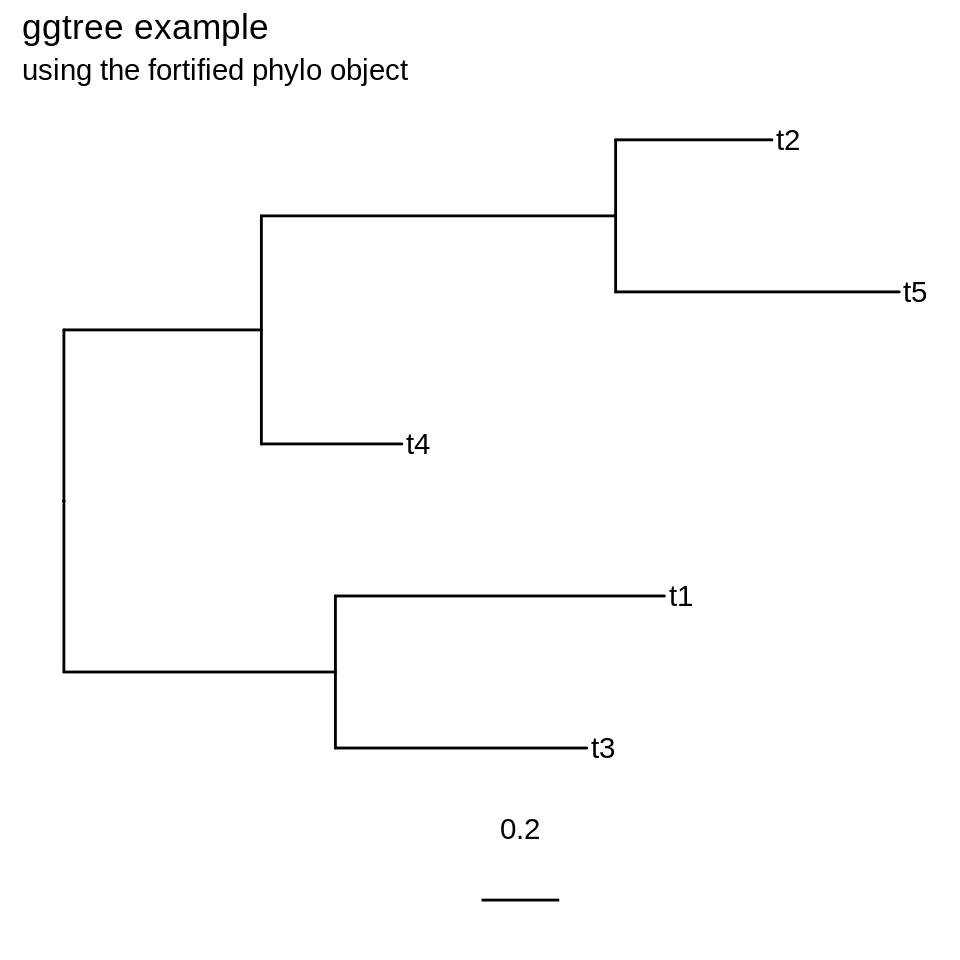The object created by `ggtree` is still a ggplot object and still can be used as such. We can use the phylo object with other geom on top of the tree. I added `geom_line`, no need to change the aesthetic mapping, we can use the mapping used by the tree. I also added `geom_point`, here I didn’t needed to modify the mapping but just to show how we can use another variable from the data set for just one geom.

You can notice that `geom_line` also have a size parameter. But this parameter is not mapped to any variable and stays identical for every line drawn. On the other hand the `size` of the points is inside an aesthetic mapping. If you look at the previous table and look at the values of the variable `angle` you can see it ranges from 72 to 360, but that’s not the values used by the geom. These values are scaled and we would need to use `scale_size_continuous` to adjust the size of the dots from the data.

``````ggtree(tr = random_tree) +
geom_tiplab() +
geom_treescale() +
geom_line(colour = "#e41a1c", size = 2) +
geom_point(mapping = aes(size = angle), colour = "#4daf4a")
``````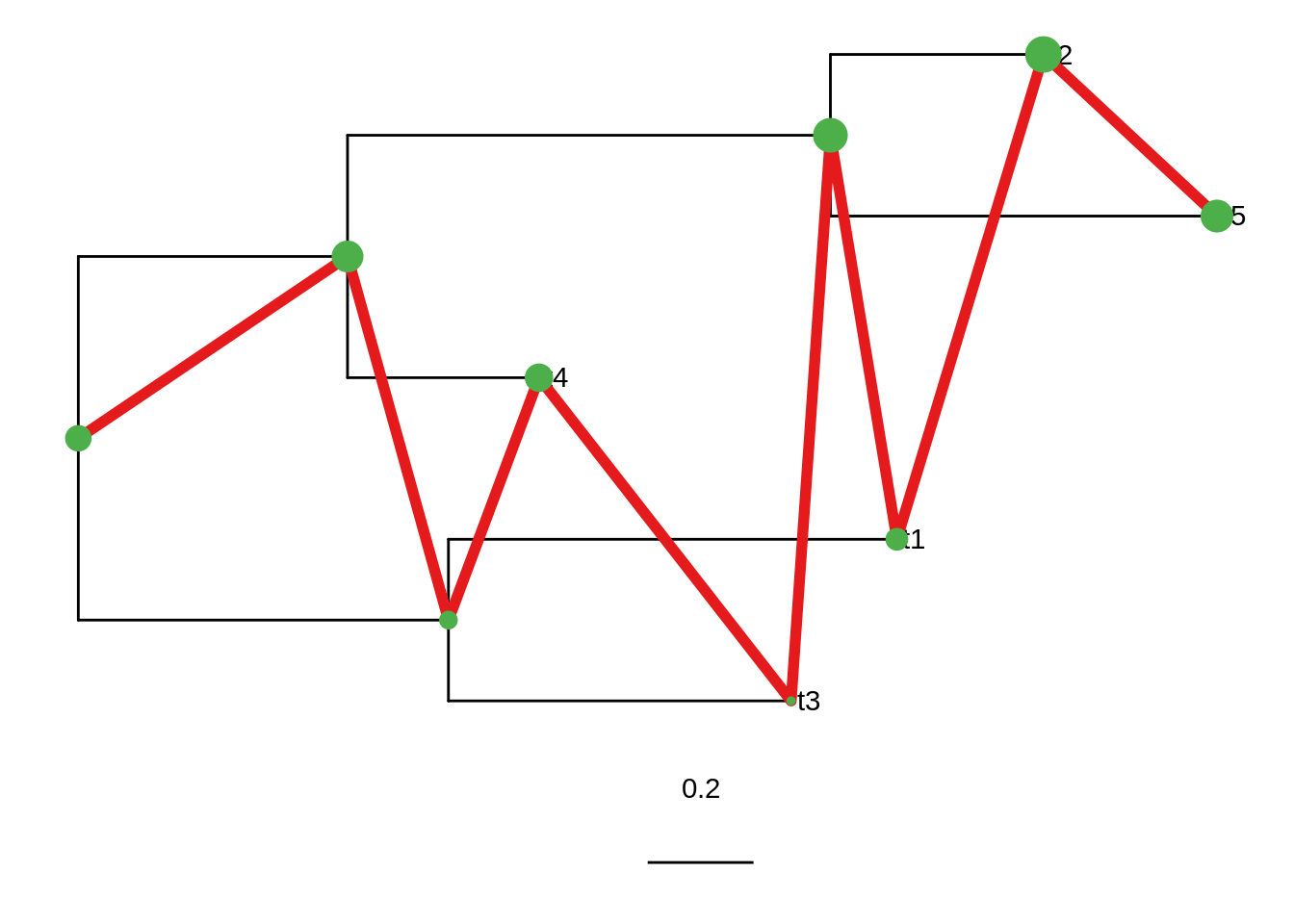More examples and documentation is available from the official website: ggtree.

Next blog posts: In the next blog posts I will talk about how to annotate and use the data structure of ggplot2/ggtree to our advantage, how to visualise more data on a tree and how to use information from the tree to create other visualisations. I will focus on examples not covered by the documentation and try to give some tricks and little hacks to programmatically annotate trees with a second data set.

PS: I want to thanks the “spelling” and “hunspell” developers whose work helped me catch a lot of typos. I’m sure there are grammar errors I missed, please don’t hesitate to send me feedback.

1. Yu, G., Smith, D. K., Zhu, H., Guan, Y. and Lam, T. T.-Y. (2017), ggtree: an r package for visualization and annotation of phylogenetic trees with their covariates and other associated data. Methods Ecol Evol, 8: 28–36. doi: 10.11112041-210X.12628 ^
2. R and ggplot2 use HTML/CSS colours, the hexadecimal notation or the colour name both work ^
3. please don’t leave now, we are going to plot something I promise! ^
4. yes, I know I also was surprised when I discovered that. ^
5. Do not despair. ^